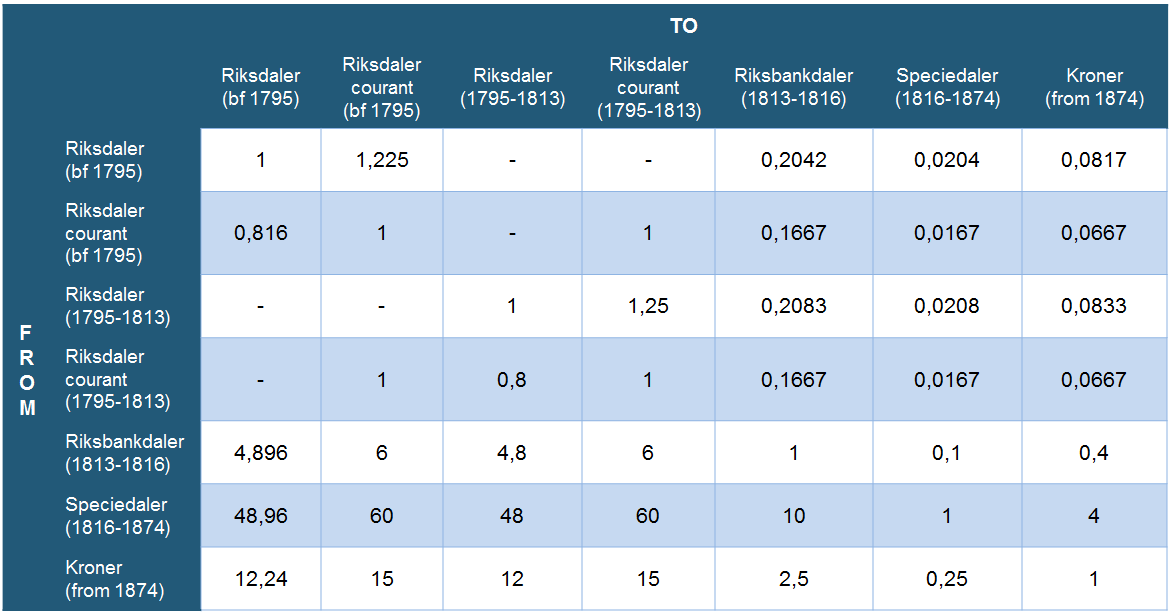# Price calculator

The price calculator allows you to calculate the equivalent value of 100 1900-kroner in 2003, or the corresponding value of 3000 1990-kroner in 1816.

## Calculate price inflation

= NOK*

*You can calculate price inflation from 1516 up to last year, but note that the result is expressed in kroner for all years back to 1516, even though the krone wasn’t introduced until 1874.

## On the use of the price calculator when transitioning between different monetary units

When using the price calculator, make sure that the starting amount is stated in the monetary unit you want to calculate inflation in. The conversion between the different monetary units in Norway from 1514 is done by multiplying with an adjustment factor according to the following table:### Examples:

If you want to calculate inflation of 100 riksdaler from year 1800 to kroner in year 2014, do the following conversion: 100 * 0.0833 = 8.33. You then enter 8.33 in the field for amount and select from the year 1800 to the year 2014. The calculator then calculates the amount in 2014-kroner, adjusted for inflation over the period 1800-2014.

If you want to calculate inflation of 100 riksdaler from year 1800 to speciedaler in 1860, do the following conversion: 100 * 0.0208 = 2.08. You then enter 2.08 in the field for amount and select from the year 1800 to the year 1860. The calculator then calculates amounts in 1860 speciedaler adjusted for inflation over the period 1800-1860.